This book is archived and will be removed July 6, 2022. Please use the updated version.

Electric Potential

# 45 Determining Field from Potential

### Learning Objectives

By the end of this section, you will be able to:

• Explain how to calculate the electric field in a system from the given potential
• Calculate the electric field in a given direction from a given potential
• Calculate the electric field throughout space from a given potential

Recall that we were able, in certain systems, to calculate the potential by integrating over the electric field. As you may already suspect, this means that we may calculate the electric field by taking derivatives of the potential, although going from a scalar to a vector quantity introduces some interesting wrinkles. We frequently need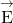to calculate the force in a system; since it is often simpler to calculate the potential directly, there are systems in which it is useful to calculate V and then derivefrom it.

In general, regardless of whether the electric field is uniform, it points in the direction of decreasing potential, because the force on a positive charge is in the direction ofand also in the direction of lower potential V. Furthermore, the magnitude ofequals the rate of decrease of V with distance. The faster V decreases over distance, the greater the electric field. This gives us the following result.

Relationship between Voltage and Uniform Electric Field

In equation form, the relationship between voltage and uniform electric field is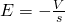where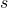is the distance over which the change in potential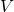takes place. The minus sign tells us that E points in the direction of decreasing potential. The electric field is said to be the gradient (as in grade or slope) of the electric potential.

For continually changing potentials,andbecome infinitesimals, and we need differential calculus to determine the electric field. As shown in (Figure), if we treat the distanceas very small so that the electric field is essentially constant over it, we find that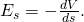The electric field component along the displacementis given by. Note that A and B are assumed to be so close together that the field is constant along.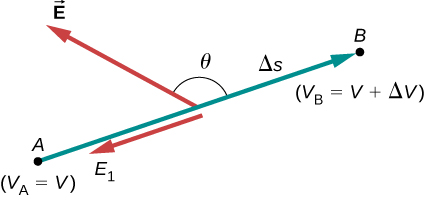Therefore, the electric field components in the Cartesian directions are given by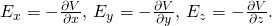This allows us to define the “grad” or “del” vector operator, which allows us to compute the gradient in one step. In Cartesian coordinates, it takes the form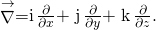With this notation, we can calculate the electric field from the potential with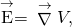a process we call calculating the gradient of the potential.

If we have a system with either cylindrical or spherical symmetry, we only need to use the del operator in the appropriate coordinates: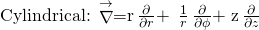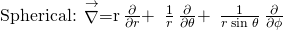Electric Field of a Point Charge Calculate the electric field of a point charge from the potential.

Strategy The potential is known to be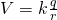, which has a spherical symmetry. Therefore, we use the spherical del operator in the formula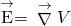.

Solution Performing this calculation gives us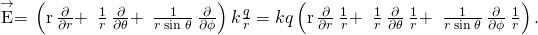This equation simplifies to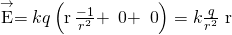as expected.

Significance We not only obtained the equation for the electric field of a point particle that we’ve seen before, we also have a demonstration thatpoints in the direction of decreasing potential, as shown in (Figure).

Electric field vectors inside and outside a uniformly charged sphere.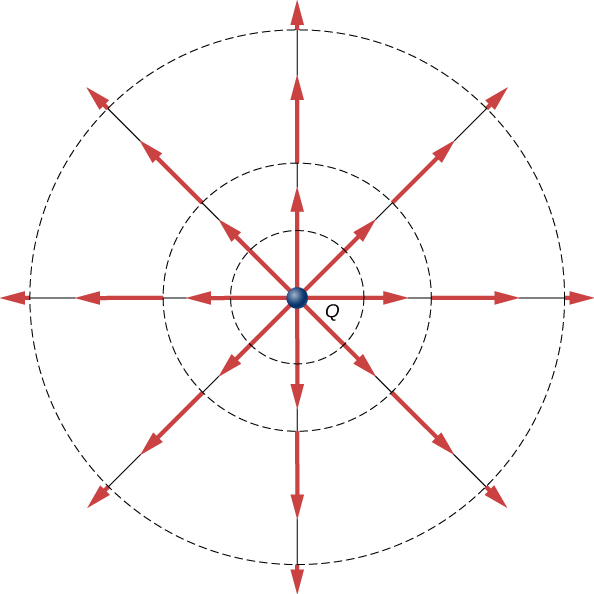Electric Field of a Ring of Charge Use the potential found in (Figure) to calculate the electric field along the axis of a ring of charge ((Figure)).

We want to calculate the electric field from the electric potential due to a ring charge.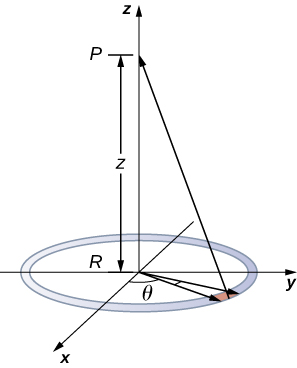Strategy In this case, we are only interested in one dimension, the z-axis. Therefore, we use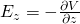with the potential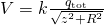found previously.

Solution Taking the derivative of the potential yields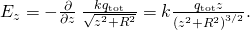Significance Again, this matches the equation for the electric field found previously. It also demonstrates a system in which using the full del operator is not necessary.

Check Your Understanding Which coordinate system would you use to calculate the electric field of a dipole?

Any, but cylindrical is closest to the symmetry of a dipole.

### Summary

• Just as we may integrate over the electric field to calculate the potential, we may take the derivative of the potential to calculate the electric field.
• This may be done for individual components of the electric field, or we may calculate the entire electric field vector with the gradient operator.

### Conceptual Questions

If the electric field is zero throughout a region, must the electric potential also be zero in that region?

No. It will be constant, but not necessarily zero.

Explain why knowledge of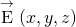is not sufficient to determine V(x,y,z). What about the other way around?

### Problems

Throughout a region, equipotential surfaces are given by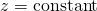. The surfaces are equally spaced with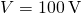for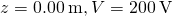for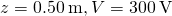for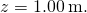What is the electric field in this region?

The problem is describing a uniform field, so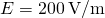in the –z-direction.

In a particular region, the electric potential is given by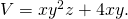What is the electric field in this region?

Calculate the electric field of an infinite line charge, throughout space.

Applywith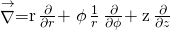to the potential calculated earlier,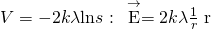as expected.